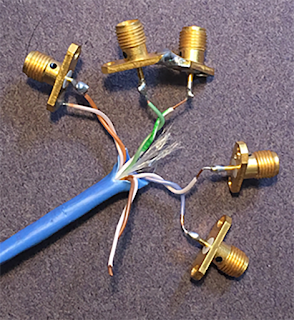## You need to test, we're here to help.

You need to test, we're here to help.

## 24 August 2020

### How to Connect the Returns to an Unshielded Twisted PairFigure 1. Three options for connecting a TDR to a UTP.
During a recent webinar, Dr. Eric Bogatin was asked several questions about how to measure unshielded twisted pairs (UTPs), which are differential pairs with no return path. Here is his answer to one of the questions. For more information, check out the whole webinar on Differential Pairs with No Return Paths.

Q: For the UTP measurement, do you need to connect the SMA connector shield grounds together?

A: Yes. It’s very important to make sure the grounds are connected at the cable connectors.

There are really three ways the connections can be made from a differential TDR to the UTP cable. These three options are shown clockwise from left to right in Figure 1.

1. Connect the two wires as a signal and return to one SMA. This treats the differential pair as a single-ended transmission line. We would expect that a single-ended measurement in this case would be the same impedance as the differential impedance, as measured by a 2-port set up.
2. Connect the two return paths at the SMAs.
3. Keep the two returns of the SMA separated.

In principle, it should be OK to keep the returns isolated or connected. After all, once the signal is launched into the twisted pair, it’s all about the UTP cable.

In practice, though, what we are really doing is launching a single-ended signal into first one line, measuring all the S-parameters, and then launching into the other line and measuring all the S-parameters. We combine the S-parameters to calculate the mixed mode S-parameters.  The, we convert the S-parameters into the time domain response.
This basically means we are going to subtract two big numbers, the single-ended return loss and the coupling term, to get a smaller number, the differential return loss. The result is very sensitive to the uncorrelated noise in the two measurements. The return losses are all about the huge impedance discontinuity of the signal path through each wire and the return path being so far away, plus the coupling to the adjacent wire.

By connecting the grounds together at the SMA, we reduce the stray fields from the two cables of the TDR, and thus are able to get less noise and a more stable measurement. When we subtract the two big numbers to get the differential return loss, it is a cleaner result.Figure 2. Measured TDR response of  UTP.
Using this technique, we are able to get very clean, very reproducible results that make a lot of sense. Figure 2 shows the measured TDR response from the UTP. In this example, the top, blue trace is the single-ended impedance of one wire in the UTP, with the other wire connected to the TDR, but just terminated. This is the single-ended response with a 50 Ohm resistor in series with the second line’s impedance. This impedance is about 100 Ohms + 50 Ohms.

The middle trace in green, is the single-ended response of the two wires treated as a single-ended transmission line, in a single-ended TDR measurement, using both wires soldered to the same SMA. This is the first configuration in Figure 1. This treats the two wires twisted together as a single-ended transmission line. The impedance is about 100 Ohms. This is the same as the differential impedance of the pair.

In the third case, in teal, the two wires are driven with a differential signal, but the TDR response of just one line is measured. This is the odd mode impedance of one line that is part of the pair. The odd mode impedance is 50 Ohms. We would expect the differential impedance of the pair to be 100 Ohms. This is exactly what we measure with the single-ended measurement.